# Python金融大数据分析-数据获取与简单处理

Python的功能不可以说不大，在金融数据分析里面有着很方便的应用。

# 1.数据获取

import numpy as np
import pandas as pd
import pandas.io.data as web
import math
#从雅虎财经获取DAX指数的数据
DAX = web.DataReader(name='^GDAXI', data_source='yahoo',start = '2000-1-1')
#查看一下数据的一些信息 上面这一方法返回的是一个pandas dataframe的数据结构
print DAX.info()
#绘制收盘价的曲线
DAX['Close'].plot(figsize=(8,5))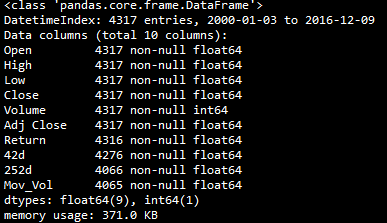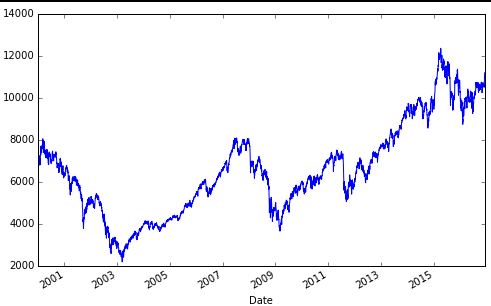# 2.简单的数据处理

#计算每日的涨跌幅
DAX['Return'] = np.log(DAX['Close']/DAX['Close'].shift(1))
print DAX[['Close','Return']].tail()
#将收盘价与每日涨跌幅度放在一张图上
DAX[['Close','Return']].plot(subplots = True,style = 'b',figsize=(8,5))
#42与252个交易日为窗口取移动平均
DAX['42d']=pd.rolling_mean(DAX['Close'],window=42)
DAX['252d']=pd.rolling_mean(DAX['Close'],window=252)
#绘制MA与收盘价
DAX[['Close','42d','252d']].plot(figsize=(8,5))
#计算波动率，然后根据均方根法则进行年化
DAX['Mov_Vol']=pd.rolling_std(DAX['Return'],window = 252)*math.sqrt(252)
DAX[['Close','Mov_Vol','Return']].plot(subplots = True, style = 'b',figsize = (8,7))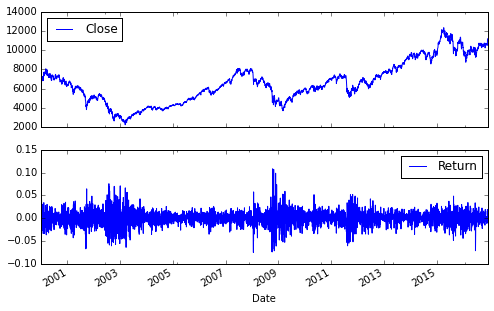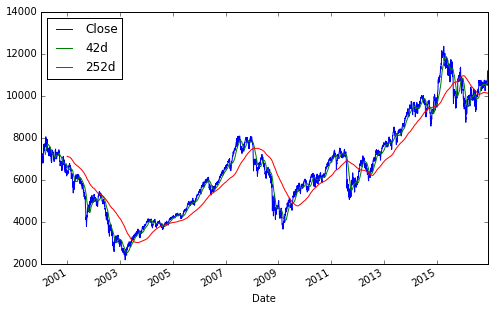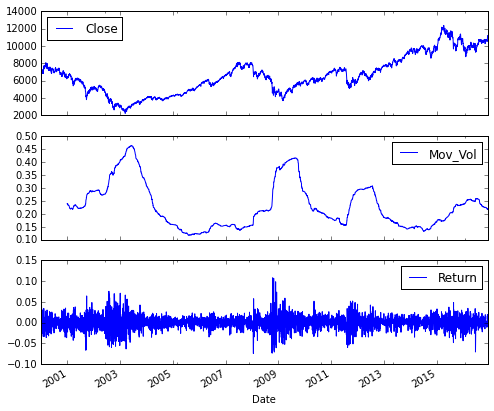这是市场的波动率和股市的关系。和FRM中提到的一样，在市场低迷，或者说，金融危机的时候，市场的波动率急剧增加。于是，就有了恐慌指数这个东西，也就是Vix，其实就是市场的波动率指数。